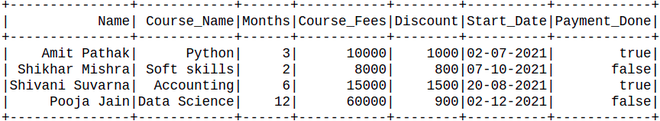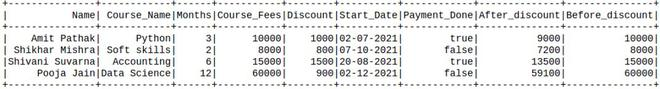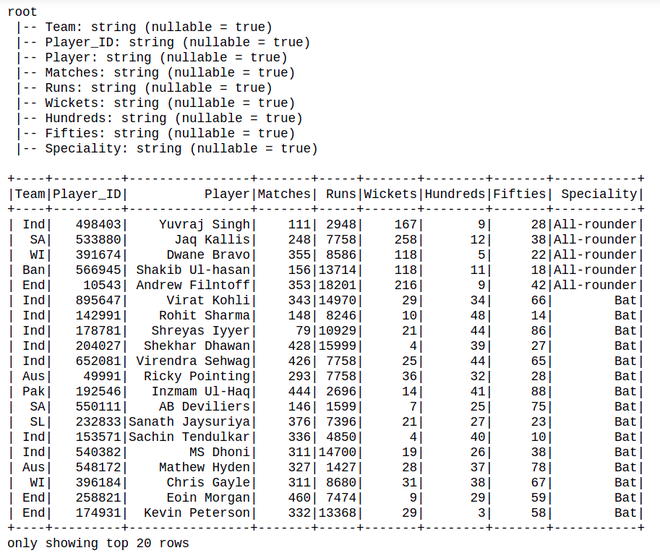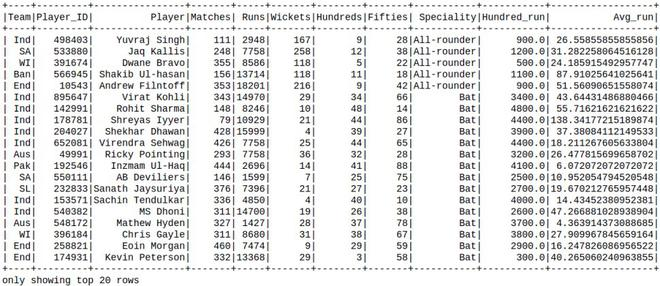# Adding two columns to existing PySpark DataFrame using withColumn

• Last Updated : 23 Aug, 2021

In this article, we are going to see how to add two columns to the existing Pyspark Dataframe using WithColumns.

WithColumns is used to change the value, convert the datatype of an existing column, create a new column, and many more.

Attention geek! Strengthen your foundations with the Python Programming Foundation Course and learn the basics.

To begin with, your interview preparations Enhance your Data Structures concepts with the Python DS Course. And to begin with your Machine Learning Journey, join the Machine Learning - Basic Level Course

Syntax: df.withColumn(colName, col)

Returns: A new :class:`DataFrame` by adding a column or replacing the existing column that has the same name.

Example 1: Creating Dataframe and then add two columns.

Here we are going to create a dataframe from a list of the given dataset.

## Python3

 `# Create a spark session``from` `pyspark.sql ``import` `SparkSession``spark ``=` `SparkSession.builder.appName(``'SparkExamples'``).getOrCreate()`` ` `# Create a spark dataframe``columns ``=` `[``"Name"``, ``"Course_Name"``,``           ``"Months"``,``           ``"Course_Fees"``, ``"Discount"``,``           ``"Start_Date"``, ``"Payment_Done"``]``data ``=` `[``    ``(``"Amit Pathak"``, ``"Python"``, ``3``, ``10000``, ``1000``,``     ``"02-07-2021"``, ``True``),``    ``(``"Shikhar Mishra"``, ``"Soft skills"``, ``2``,``     ``8000``, ``800``, ``"07-10-2021"``, ``False``),``    ``(``"Shivani Suvarna"``, ``"Accounting"``, ``6``,``     ``15000``, ``1500``, ``"20-08-2021"``, ``True``),``    ``(``"Pooja Jain"``, ``"Data Science"``, ``12``,``     ``60000``, ``900``, ``"02-12-2021"``, ``False``),``]`` ` `df ``=` `spark.createDataFrame(data).toDF(``*``columns)`` ` `# View the dataframe``df.show()`

Output:Here, we create two-column based on the existing columns.

## Python3

 `new_df ``=` `df.withColumn(``'After_discount'``,``                       ``df.Course_Fees ``-` `df.Discount).withColumn(``'Before_discount'``,``                                                                ``df.Course_Fees)``new_df.show()`

Output:Example 2: Creating Dataframe from csv and then add the columns.

Here we will use the cricket_data_set_odi.csv file as a dataset and create dataframe from this file.

Creating Dataframe for demonstration:

## Python3

 `# import pandas to read json file``import` `pandas as pd`` ` `# importing module``import` `pyspark`` ` `# importing sparksession from pyspark.sql``# module``from` `pyspark.sql ``import` `SparkSession`` ` `# creating sparksession and giving an app name``spark ``=` `SparkSession.builder.appName(``'sparkdf'``).getOrCreate()`` ` ` ` `# create Dataframe``df ``=` `spark.read.option(``"header"``,``True``).csv(``"Cricket_data_set_odi.csv"``)`` ` `# Display Schema``df.printSchema()`` ` `# Show Dataframe``df.show()`

Output:Then, Adding the columns in an existing Dataframe:

## Python3

 `new_df ``=` `df.withColumn(``    ``'Hundred_run'``, df.Hundreds``*``100``).withColumn(``    ``'Avg_run'``, df.Runs ``/` `df.Matches)`` ` `new_df.show()`

Output:My Personal Notes arrow_drop_up